Latest Banking jobs   »   Basic Quantitative Aptitude Quiz for All...

# Basic Quantitative Aptitude Quiz for All Banking Exams- 19th May

Q1. In how many different ways the letters of word ‘DANGER’ can be arranged?
(a) 5040
(b) 640
(c) 720
(d)120
(e) 520

Q2. Find the probability of selecting 2 green balls out of 4 green and 3 red balls.
(a) 6/7
(b) 4/7
(c) 3/7
(d) 2/7
(e) 1/7

Q3. The ratio between length and breadth of a rectangular field is 3 : 2. If perimeter of field is 250. Find the area of rectangular field.
(a) 3750 sq. unit
(b) 3570 sq. unit
(c) 3650 sq. unit
(d) 3250 sq. unit
(e) 3950 sq. unit

Q4. A bag contains 5 blue balls and 7 red balls, if 2 balls are drawn at random. What is the probability that at least 1 ball is red.
(a) 28/33
(b) 17/66
(c) 23/33
(d) 47/66
(e) 43/66

Q5. Radius of a circular ring is 21 cm. If this ring is folded in square form, then find the length of diagonal of square.
(a) 25 cm
(b) 33√2 cm
(c) 28 cm
(d) 35√3 cm
(e) 32√3 cm

Q6. In how many ways can the letters of word ‘NOUVEAU’ can be arranged.
(a) 1840
(b) 1260
(c) 5040
(d) 2520
(e) 2240

Q7. A dice is thrown up find the probability of getting an odd no. on the upper face.
(a) 3/4
(b) 2/3
(c) 1/2
(d) 5/6
(e) 1/3

Q8. How many five digits number can be formed using the digits 1, 3, 4, 5, 6, 7 without repetition of digits?
(a) 120
(b) 144
(c) 720
(d) 840
(e) 420

Q9. In how many ways can a group of 5 men and 2 women be made out of total of 7 men and 3 women?
(a) 63
(b) 45
(c) 126
(d) 90
(e) 84

Q10. The ratio between perimeter of an equilateral triangle and a square is 3 : 8. The area of square is 800% of area of a rectangle having sides 8 m and 4 m. Find the perimeter of triangle.
(a) 22 m
(b) 20m
(c) 24 m
(d) 28 m
(e) 32 m

Q11. A bag has seven red, four white and three green balls while another bag has five red, six yellow and three blue balls. A bag is selected at random and a ball drawn out of it, then Find the probability that the ball drawn is red.
(a) 1/7
(b) /7
(c) 2/7
(d) 1
(e) 6/7

Q12. Find the ratio of volume of cylinder ‘A’ to volume of cylinder ‘B’, if ratio of radius of cylinders ‘A’ to cylinder ‘B’ is 1 : 2 while the ratio of height of cylinder ‘A’ to cylinder ‘B’ is 2 : 1.
(a) 1 : 1
(b) 1 : 2
(c) 2 : 1
(d) 1 : 4
(e) 1 : 8

Q13. Find the ratio of area of a circle to area of square if perimeter of circle and square is equal?
(a) 11 : 14
(b) 11 : 4
(c) 8 : 11
(d) 14 : 11
(e) 2 : 4

Q14. There are 4 Red, 2 Green & 2 Blue ball in a bag. If one ball drawn at random from the bag, then find the probability of the ball drawn out is not blue?
(a) 1/4
(b) 3/8
(c) 3/6
(d) 5/8
(e) 3/4

Q15. A father has 3 children with at least one boy. The probability that he has 2 boy and one girls
(a) 1/4
(b) 1/3
(d) 2/3
(d) 3/8
(e) 5/8

Practice More Questions of Quantitative Aptitude for Competitive Exams:

Solutions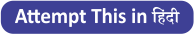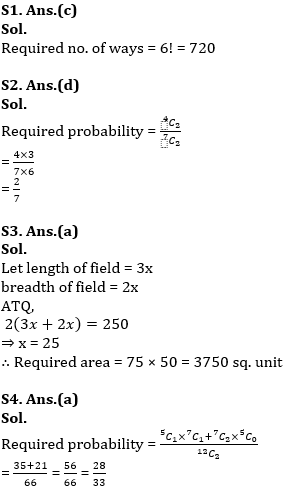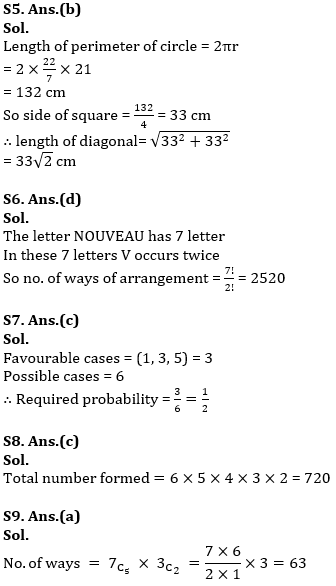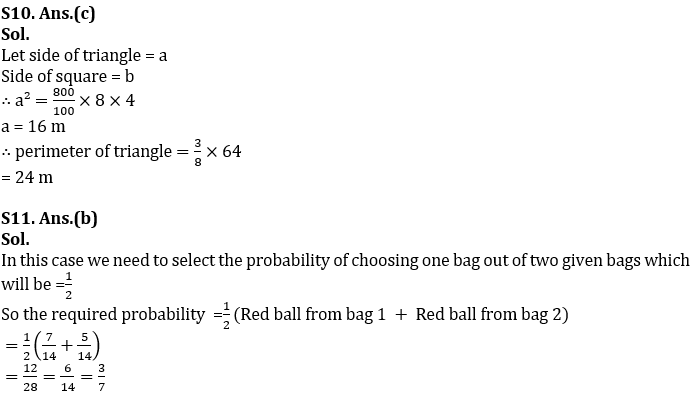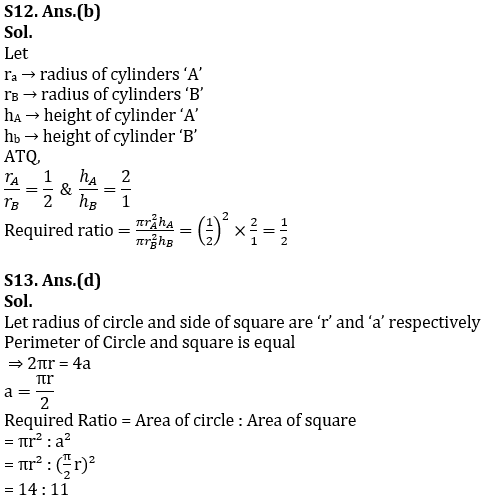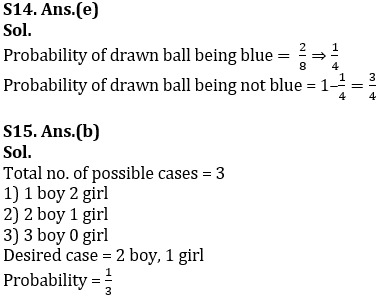#### Congratulations!Incorrect details? Fill the form again here

•Reasoning Ability Quiz For IBPS RRB PO C...
•Quantitative Aptitude Quiz For IBPS RRB ...
•Important Current Affairs Quiz for Bank ...
•Reasoning Ability Quiz For IBPS Clerk Pr...
•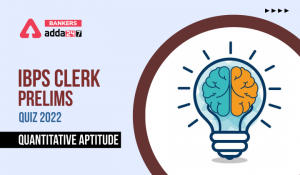Quantitative Aptitude Quiz For IBPS Cler...
•English Quizzes For IBPS Clerk Prelims 2...